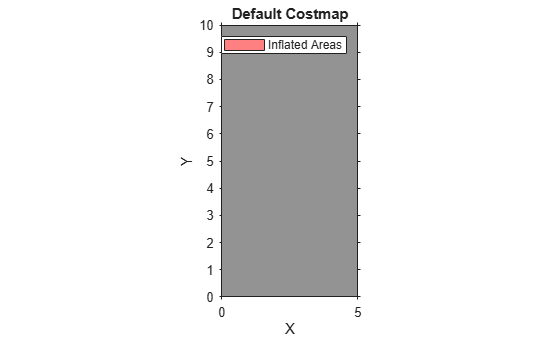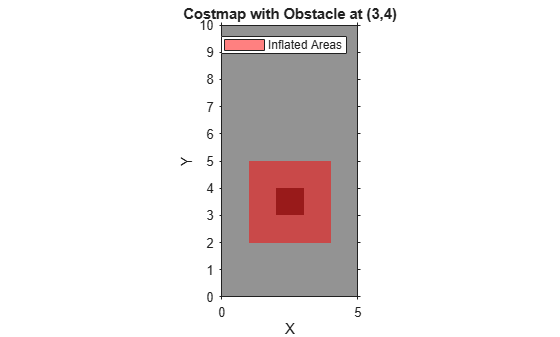# getCosts

Get cost value of cells in vehicle costmap

## Syntax

``costVals = getCosts(costmap,xyPoints)``
``costMat = getCosts(costmap)``

## Description

example

````costVals = getCosts(costmap,xyPoints)` returns a vector, `costVals`, that contains the costs for the (x, y) points in `xyPoints` in the vehicle costmap.```
````costMat = getCosts(costmap)` returns a matrix, `costMat`, that contains the cost of each cell in the costmap.```

## Examples

collapse all

Create a 5-by-10 meter vehicle costmap. Cells have side length 1, in the world units of meters. Set the inflation radius to 1. Plot the costmap, and get the default cost matrix.

```costmap = vehicleCostmap(5,10); costmap.CollisionChecker.InflationRadius = 1; plot(costmap) title('Default Costmap')````getCosts(costmap)`
```ans = 10×5 0.4250 0.4250 0.4250 0.4250 0.4250 0.4250 0.4250 0.4250 0.4250 0.4250 0.4250 0.4250 0.4250 0.4250 0.4250 0.4250 0.4250 0.4250 0.4250 0.4250 0.4250 0.4250 0.4250 0.4250 0.4250 0.4250 0.4250 0.4250 0.4250 0.4250 0.4250 0.4250 0.4250 0.4250 0.4250 0.4250 0.4250 0.4250 0.4250 0.4250 0.4250 0.4250 0.4250 0.4250 0.4250 0.4250 0.4250 0.4250 0.4250 0.4250 ```

Mark an obstacle at the (x,y) coordinate (3,4) by increasing the cost of that cell.

```setCosts(costmap,[3,4],0.8); plot(costmap) title('Costmap with Obstacle at (3,4)')```Get the cost of three cells: the cell with the obstacle, a cell adjacent to the obstacle, and a cell outside the inflation radius of the obstacle.

`costVal = getCosts(costmap,[3 4;2 4;4 7])`
```costVal = 3×1 0.8000 0.4250 0.4250 ```

Although the plot of the costmap displays the cell with the obstacle and its adjacent cells in shades of red, only the cell with the obstacle has a higher cost value of 0.8. The other cells still have the default cost value of 0.425.

## Input Arguments

collapse all

Costmap, specified as a `vehicleCostmap` object.

Points, specified as an M-by-2 real-valued matrix that represents the (x, y) coordinates of M points.

Example: `[3.4 2.6]` specifies a single point at (3.4, 2.6)

Example: `[3 2;3 3;4 7]` specifies three points: (3, 2), (3, 3), and (4, 7)

## Output Arguments

collapse all

Cost of points in `xyPoints`, returned as an M-element real-valued vector.

Cost of all cells in `costmap`, returned as a real-valued matrix of the same size as the costmap grid. This size is specified by the `MapSize` property of the costmap.

## Version History

Introduced in R2018a